# Self-reproducing cellular automata

Edward Fredkin is best known these days for the Fredkin gate, a universal reversible circuit.

I recently found out that Fredkin was one of the pioneers in cellular automata. His student Edwin Banks describes a cellular automaton introduced by Fredkin .

Edward Fredkin of MIT has described the following interesting cellular space. If the states are 0 and 1 and if the result state is obtained by taking the sum of the neighbors, modulo 2, then every initial configuration will reproduce copies of itself about the initial configuration.

In other words, start with some bit pattern in an infinite checkerboard of bits. Then at each step, replace each bit by the parity of the sum of the neighboring bits. Eventually you’ll see copies of that original pattern.

There’s some ambiguity regarding what “neighbors” means. Banks’ dissertation considers the neighbors of a bit to be the bits to the north, south, east, and west, and this implies that Banks has these neighbors in mind when he describes Fredkin’s automaton. Other sources say Fredkin also considered diagonally adjacent bits as part of the neighborhood, i.e. northwest, northeast, southwest, and southeast.

Banks goes on to say

Terry Winograd (1970) generalized this result showing that any neighborhood, not just the four nearest neighbors, and any number of dimensions still yields the same results.

I take it by “the four nearest neighbors” the neighbors to the north, south, east, and west. I’m not sure what the “any neighborhood” means in Winograd’s theorem; I imagine there must be some implied restriction.

In any case, I’ll show by example that both definitions of “neighborhood” discussed above lead to reproducing the original pattern, though they do not reproduce it in the same way.

I’ll start with an ‘E’ in the middle of a grid. The black squares represent 0s and the white squares represent 1s.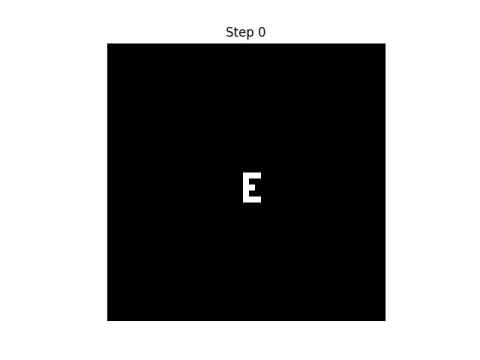Here are the first eight steps in evolving this pattern, using the rule that “neighbor” only includes north, south, east, and west neighbors.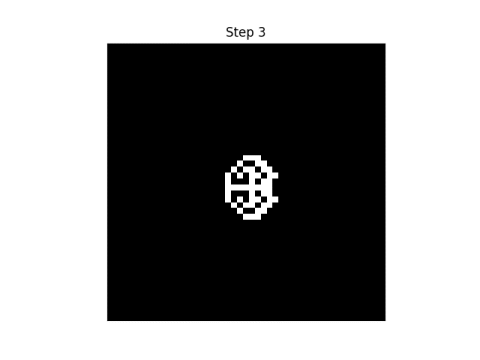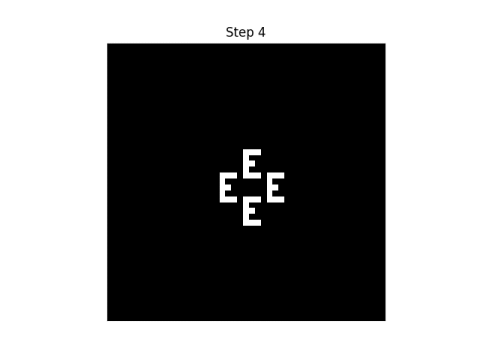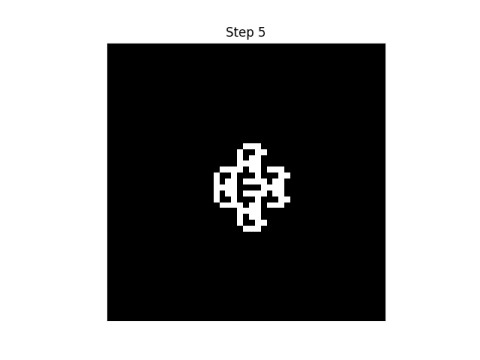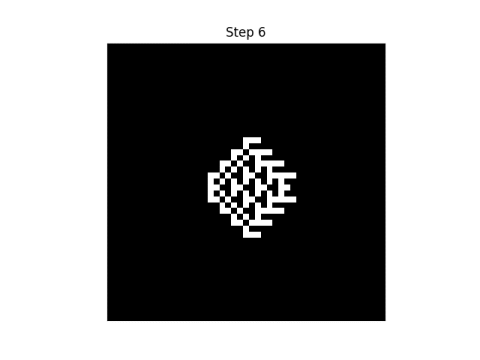If we consider each point to have eight neighbors, i.e. we include diagonals, here’s what the first eight steps look like.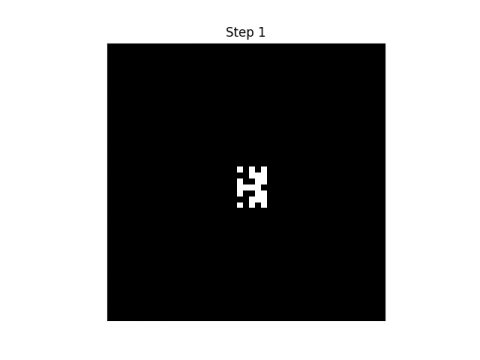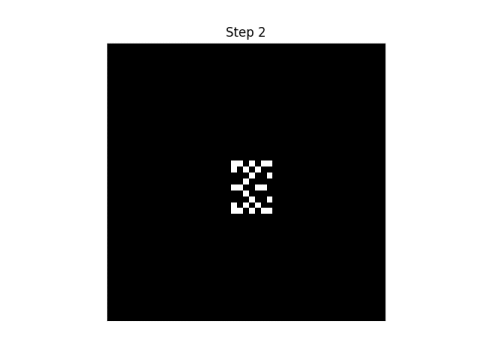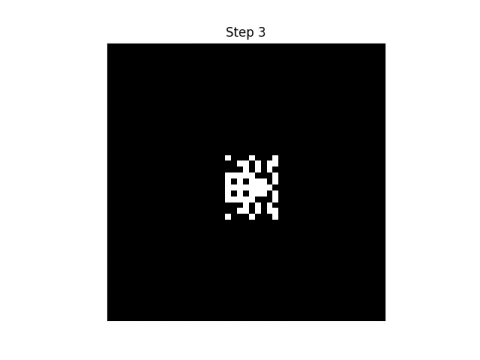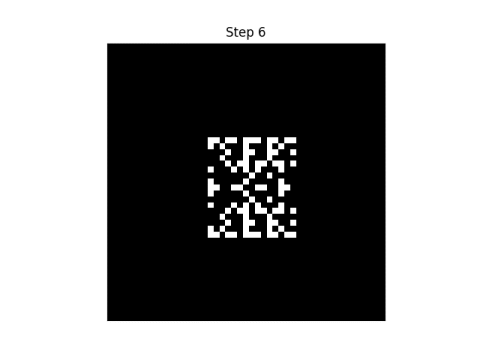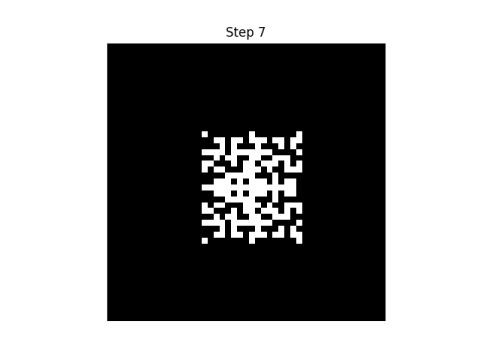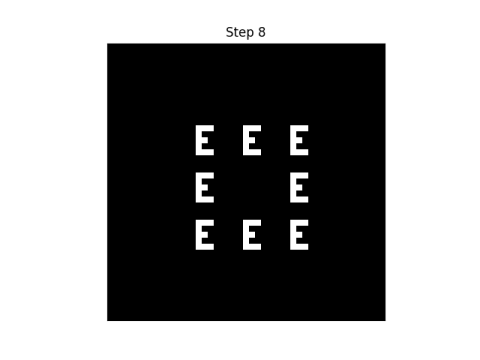Update: Here’s an animated version of the first automatonand the secondSee the next post for a generalization using more than two states per cell, using colors rather than black and white cells. If the number of colors is prime, and you take the sum of the neighboring states mod p rather than mod 2, you get analogous results.

 Edwin R. Banks, Information Processing and Transmission in Cellular Automata. MIT dissertation. January 1971.

## 7 thoughts on “Self-reproducing cellular automata”

1. Labels on animations are reversed?

2. Thanks. Fixed.

3. Jesse

These two neighbourhoods have convenient names: the one with four neighbours is the von Neumann neighbourhood and the one with eight is the Moore neighbourhood.

4. I am very curiously to this theory of cellular automata..can I have a MATLAB or python code which generate shapes?

5. Johan

The only implied restriction in Winograd’s theorem is that there must be at least two neighbors. If there is just one neighbor, then the initial configuration is just shifted in some direction.

The idea is as follows. In the one-dimensional case finite a configuration can be represented as a polynomial p(x) with coefficients modulo 2 (e.g. if there are ones at coordinates -1 and 2, this is represented by the polynomial x^(-1) + x^2). Then the action of the summation automaton can be represented as a multiplication p(x)*f(x) modulo 2 by some polynomial f(x), e.g. f(x)=x+x^3. By a theorem known as “Freshman’s dream” f(x)^n=f(x^n) modulo 2 whenever n is a power of 2, so in the previous example f(x)^(2^10) = x^1024 + x^3072. The effect of p(x)*f(x)^(2^10) is just to make (possibly overlapping) copies of the pattern p(x) at coordinates 1024 and 3072. When the size of n, the power of 2, is larger than the diameter of p(x), then the copies of p(x) in p(x)*f(x)^n do not overlap.

The multidimensional case is handled by considering polynomials in multiple variables.